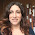## Tuesday, December 7, 2010

### What is the difference between a simulation and a model? [Updated]

by Beat Schwendimann

The terms simulation and model are often used synonymously. However, I suggest the following distinction:

• A model is a product (physical or digital) that represents a system of interest. A model is similar to but simpler than the system it represents, while approximating most of the same salient features of the real system as close as possible. A good model is a judicious tradeoff between realism and simplicity. A key feature of a model is manipulability. A model can be a physical model (for example a physical architectural house scale model, a model aircraft,  a fashion mannequin, or a model organism in biology research); or a conceptual model (for example a computer model, a statistical or mathematical model, a business model.
• Modeling is the act of building a model.
• A simulation is the process of using a model to study the behavior and performance of an actual or theoretical system. In a simulation, models can be used to study existing or proposed characteristics of a system. The purpose of a simulation is to study the characteristics of a real-life or fictional system by manipulating variables that cannot be controlled in a real system. Simulations allow evaluating a model to optimize system performance or to make predictions about a real system. Simulations are useful to study properties of a model of a real-life system that would otherwise be too complex, too large/small, too fast/slow, not accessible, too dangerous or unacceptable to engage. While a model aims to be true to the system it represents, a simulation can use a model to explore states that would not be possible in the original system.
• Simulating is the act of using a model for a simulation.

References:
-Maria, A. (1997). Introduction to modeling and simulation. In Proceedings of the 29th conference on winter simulation.

1.Thanks for posting. It helped me.

2.Thanks Beat! I am quoting you in book. :D

3.Thanks a lot sir. you solved my problem :)

4.Thanks a lot Beat. Quite informative..

5.Thank you so much. Very Nice.

6.Thank you very much...

7.thanks a lot. Helpful and simple.

8.9.10.Plain in every sense.
Thank you...

11.12.13.Insightful, thank you